## Example Questions

1 2 4 Next →

### Example Question #31 : How To Simplify An Expression

What is the simplified version of the expression: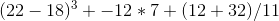?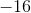Explanation:

Use PEMDAS to dictate which operation comes first. Simplify the parentheses: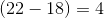and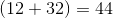.

Next come exponents: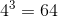After that comes multiplication and division left to right: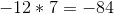and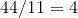.

Finally, add all the terms together: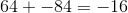### Example Question #32 : How To Simplify An Expression

The expression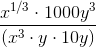can be rewritten as: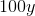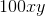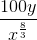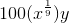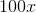Explanation:

To simplify this problem, let’s look at each term individually.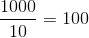;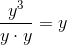;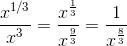. Thus B is the correct answer.

### Example Question #33 : How To Simplify An Expression

The product of two consecutive odd negative integers is. What is the smaller of the two integers?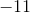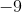Explanation:

The problem gives us the product of two consecutive odd negative integers, so we know that one number isless than the other one. Thus, we can set our two numbers asand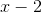.

At this point, the more algebraically inclined student might recognize that if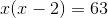, then the equation can be remade to say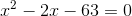, and use the quadratic formula to solve.

But this is the ACT, and the faster method by far is to simply recognize that if the product of our two integers is, thenmust be evenly divisible by our two integers. The only two choices we have that divide evenly intoareand, makingthe smaller number and our answer.

### Example Question #31 : How To Simplify An Expression

Suzanne is at the grocery store. She has $5.00 to spend on produce. Oranges are$2.50 per pound, apples cost $1.50 per pound and bananas are$0.50 per pound. Which combination of fruit will fit her budget?

1.5 pounds of oranges and 4 pounds of bananas

3 pounds of apples and 2 pounds of bananas

1 pound of oranges, 1 pound of apples and 2 pounds of bananas

1 pound of oranges, 1.5 pounds of apples and 1.5 pounds of bananas

2 pounds of oranges and 1 pound of apples

Total Cost = $2.50 * (# Oranges) +$1.50 * (# Apples) +  \$0.50 * (# Bananas)